InfoQ Homepage Articles Cats, Qubits, and Teleportation: The Spooky World of Quantum Algorithms (Part 2)

# Cats, Qubits, and Teleportation: The Spooky World of Quantum Algorithms (Part 2)

Leia em Português

### Key Takeaways

• Teleportation was one of the first quantum information processes to be described. It uses entanglement to (sort of) transmit information instantaneously across large distances. Despite the name, it can't be used to transport large objects, like people or cats.
• Quantum information theory really took off once people noticed that the computational complexity of quantum systems was actually a computational capacity, which could be applied to other problems, such as factorization, which is used within public key cryptography
• Shor’s logarithms algorithm doesn’t kill the field of cryptography; "quantum-safe" cryptography protocols are already being developed.
• Shor’s algorithm was the first quantum ‘killer app’. The second, two years later, was Grover’s search algorithm. Grover’s algorithm allows searching of an unsorted database in O(n)time.
• With the fields of both quantum computing and machine learning heating up in the past few years, it’s natural to ask if they can be combined. The answer, so far, appears to be a tentative ‘yes’.

In order to understand why quantum computers are interesting, it's necessary to (briefly!) dredge up complexity theory. Although it feels remote from what most of us do day to day, complexity theory isn't just for university courses and whiteboards in job interviews. What makes quantum computing so fast isn't the processing speed of quantum computers (which is slow) or the memory of the computers (which is absurdly tiny). Instead, it's the algorithms. Quantum computers enable new algorithms with completely different complexity characteristics than their classical equivalents.

Those who study complexity for a living classify problems into complexity classes. A given problem may belong to multiple classes, and there are so many complexity classes it's sometimes called the complexity zoo. Happily, there are only a few we need to know about. NP-hard problems are, as the name suggests, hard to solve. They get harder at an alarming rate as the number of things (digits, or points, or cities) in the solution increases. NP-complete problems are NP-hard problems whose solution can be generalised to solving any other NP-hard problem. An efficient algorithm for an NP-complete problem is the holy grail of complexity theory. (Before you get too excited, no, quantum computing doesn't offer this - yet.)

A complexity class is defined by more than its big-O notation, but in general, NP-hard problems scale polynomially with the number of things - that is, they're solvable in 2 0(log(n))time. This means a computation that takes a very reasonable 8 seconds for 3 points, would take a rather less reasonable 17 minutes for 10 points, a ridiculous 12 days for 20 points, and 35,000 years for 40 points. Another way of thinking about it is that the hardware would be obsolete before the computation finished for a 26 point calculation, and for a 31 point calculation, anyone who had written the program would most likely have died before it arrived at an answer.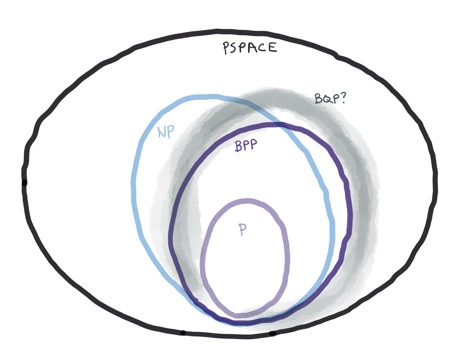## Significant quantum algorithms

### Teleportation

Although it's not a computation, no discussion of quantum information is complete without mentioning teleportation. Teleportation was one of the first quantum information processes to be described. It uses entanglement to (sort of) transmit information instantaneously across large distances. Despite the name, it can't be used to transport large objects, like people or cats. It only applies to a single particle, and it doesn't even transmit the actual particle, just information about its state. However, it can transmit the full qubit-rich state of that particle, which gets people who care about quantum states pretty excited. Quantum information cannot be transmitted via classical channels, because any classical measurement of the quantum state disrupts it (‘collapse’).

Quantum information cannot be copied (the ‘no-cloning’ theorem), so teleporting information about a particle destroys  the state of the original particle, which is probably the inspiration for the name ‘teleportation’; even though matter isn’t disintegrated in one place and regenerated in another place, information is.

Einstein's special relativity theory states that information cannot travel faster than light. Teleportation doesn't actually violate this, it just seems to (which is almost as exciting). In order to be entangled, a photon pair have to start off in the same place and then travel away from each other. This means the 'latent' information travelled slower than the speed of light, and thus respects Einstein's theory. It's a little bit like communication by carrier pigeon; although the pigeons can carry a note much quicker than a person on horseback, a horse had to transport the pigeons to their destination so that they could then be released home with a note. In order to transmit useful information (rather than just the result of a probabilistic measurement), the teleportation protocol also requires classical communication - that is, a horse has to move the carrier pigeons, and then a second horse has to follow up in the other direction with instructions for how to read the message strapped to the pigeon’s leg. This means quantum teleportation is unlikely to be used for high frequency trading in the near future. However, it’s an effective way of transmitting a complete quantum state, without collapsing it.

### Simulation of quantum systems

It’s not too surprising that a quantum system, such as a quantum computer, could be used to simulate another quantum system. What is surprising is that classical computers are pretty bad at simulating quantum systems. Small quantum systems are fine, but large ones are effectively impossible; the information richness of quantum states means the effort of doing a simulation scales exponentially with the number  of elements. Richard Feynman was the first to notice that quantum systems had seriously high computational complexity, which laid the groundwork for the later developments of quantum information theory.

### Factorization

Quantum information theory really took off once people noticed that the computational complexity of quantum systems was actually a computational capacity, which could be applied to other problems. Factorization is the problem of finding two numbers which, together, multiply to exactly make a given number. Factoring is occasionally useful for its own sake, but the main reason it’s interesting is that it’s hard, and asymmetrically hard. Factoring is an example of what’s known as a one-way function; if both divisors are known, finding their product is easy, but finding the divisors from the product can take a long time. The largest number ever factored was a 232 digit number, which really isn’t very big. (For comparison, pi has been calculated to at least five trillion digits). Factoring even 232 digits was a major project; it used hundreds of machines, and it took researchers two years to find the divisors.

This asymmetric difficulty makes factoring useful for public key cryptography (for example, RSA). The product of two primes is used to generate a public key, which senders use to encrypt a message. The recipient then uses their private key, based on one of the original factors, to decrypt the message. Decrypting if one knows the original divisors is easy; without them, it’s an intractable problem.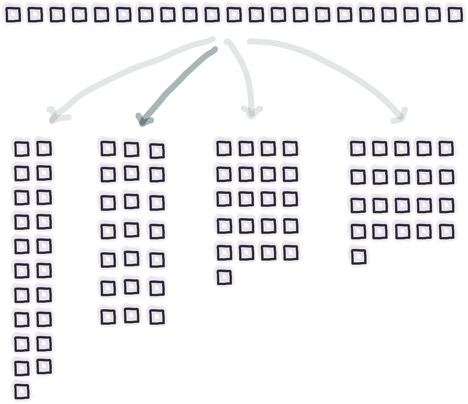Although it hasn’t been mathematically proved, it’s generally believed that the classical difficulty of the general-case factorization problem scales with the number of digits in a way that’s harder than polynomial, but easier than exponential. This is known as sub-exponential, and written as 2O(n).In practice, sufficiently large numbers are essentially un-factorable by classical means.

In 1994 Peter Shor, a mathematician, showed that a quantum computer could be used to solve the factorization problem in polynomial time. He’d heard a talk on the theoretical prospects of quantum computation, but at the time no one had identified any useful algorithms for them. He started thinking about how to make a quantum computer solve general computational problems, but didn’t tell anybody what he was doing. It took him a year of (part-time) work to come up with a quantum algorithm for finding logarithms. Four days later, he had managed to adapt his logarithms algorithm to an efficient factoring one.

Since factoring is a core part of most public-key cryptography, Shor’s work made people sit up and take notice. However, Shor’s algorithm doesn’t kill the field of cryptography; "quantum-safe" cryptography protocols are already being developed. Furthermore, as we’ll see in Part 3 of this series, it would require a quantum computer with thousands of qubits to successfully run Shor's algorithm.

Shor’s algorithm works by using quantum superposition to try out a great many possibilities.

However, the details of Shor’s algorithm are more complicated than just ‘trying out all the possible factors and seeing which one is right.’ Although this could be done on a quantum computer, any measurement would give a random - and most likely incorrect - factor.

The cleverness of Shor’s algorithm is that after trying out lots of possibilities, it then interferes all the answers together, so that a measurement is much more likely to yield a correct answer than an incorrect answer. ‘Interfering answers’ isn’t something that makes sense for a classical computer, but quantum computers take advantage of the wave-y characteristics of quantum states to do this. Interfering possibilities to amplify the correct ones is a common feature of many quantum algorithms.

The first part of Shor’s  solution is to do a classical computation to reduce the factorisation problem to one of finding the period of a function (that is, its frequency). Once that’s done, the problem is about waves and frequencies, and it starts to feel more natural that there might be a solution involving quantum waves and interference.

In classical mathematics, a Fourier transform is used to turn a function (like a wave signal) into its constituent frequencies.  To find the Fourier transform of an unknown function, it would be necessary to measure it at many points, in order to work out how often it repeated. For example, the Fourier transform of a standard sine wave is a pair of spikes (there’s only a single frequency, mirrored around the y-axis). The Fourier transform of a box function is a gradually subsiding bump.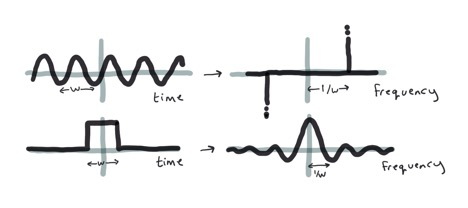The quantum Fourier transform has the same goal of picking out the frequencies which make up a timeseries, but it’s able to use superposition to effectively measure the function at multiple points in time, and then interfere the waves so that the right answers amplify, and the wrong answers cancel out. Once this is done, any measurement has a high probability of collapsing the system to the correct answer.

Mixing together waves so that wrong answers cancel themselves out is very different from how classical computers work, but it is something many of us have experienced in the macroscopic world. For example, noise cancelling headphones work by adding extra noise to existing noise. They play an extra soundwave which attempts to reproduce external noise, but with the phase shifted. As long as the new soundwaves are exactly 180 degrees out of phase with the unwanted noise waves, and a perfect copy of them, they will neatly cancel one another out, resulting in blissful silence for the wearer.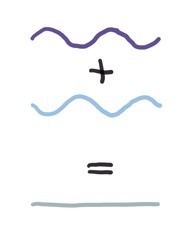### Search

Shor’s algorithm was the first quantum ‘killer app’. The second, two years later, was Grover’s search algorithm. Grover’s algorithm allows searching of an unsorted database in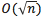time. For example, it could be used to find a name in a phone book, if one only knows the person’s phone number. Grover’s algorithm doesn’t give the same spectacular speedup as Shor’s factoring algorithm; it’s a quadratic speedup, rather than an exponential speedup (that is, it scales as the square root of the number of elements). The more modest speed-up is counter-balanced by the broad applicability of Grover’s algorithm.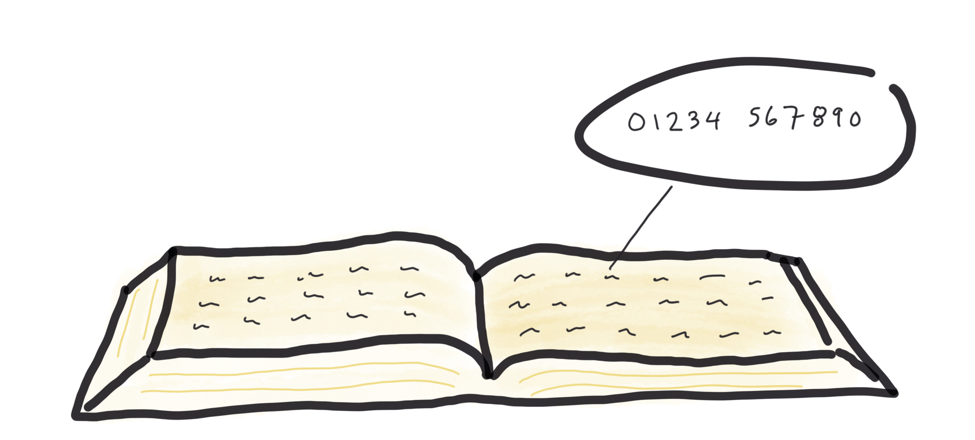Although Grover described his algorithm in terms of database search, it is in fact more general than that. The thing being searched for is something which satisfies a function - in other words, the answer to a problem. (Technically speaking, the goal is the ‘inverse of a function’.) That problem can, for example, be ‘what key should I use to decrypt this message?’ In other words, Grover’s search algorithm can be used to speed up the brute-force cracking of cryptography, even cryptography not based on factoring. It can also be used to estimate the average (mean or median) of a set of numbers.

Grover’s algorithm, like many quantum algorithms, is probabilistic. Each execution has a chance of giving the correct answer, and a - lower - chance of getting the wrong answer. This seems pretty unsatisfactory, but it’s actually more useful than it seems. Because the answer is the solution to a function, it will be immediately obvious if the algorithm gave the wrong answer. For an n-element system, having spenttime working away on a solution, only to get the wrong answer, would be pretty depressing. However, even if the long slow calculation has to be repeated several times, the total solution time is still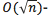and much faster than it would be using a deterministic classical algorithm. (The probability of the calculation being wrong does not increase with n, which is why the chance of error is tolerable.)

### Collision

A generalisation of Grover's algorithm can be used to solve ‘collision problems’. That is, instead of looking for one thing which satisfies a function, it can find multiple elements which yield the same answer. This is useful for understanding physical collisions in space, and also for more general problems, such as finding two people who share a birthday. As with Grover’s original algorithm, it can form the basis of a cryptographic attack by finding two numbers with the same hash (the classical version of this has the cheerful name ‘birthday attack’).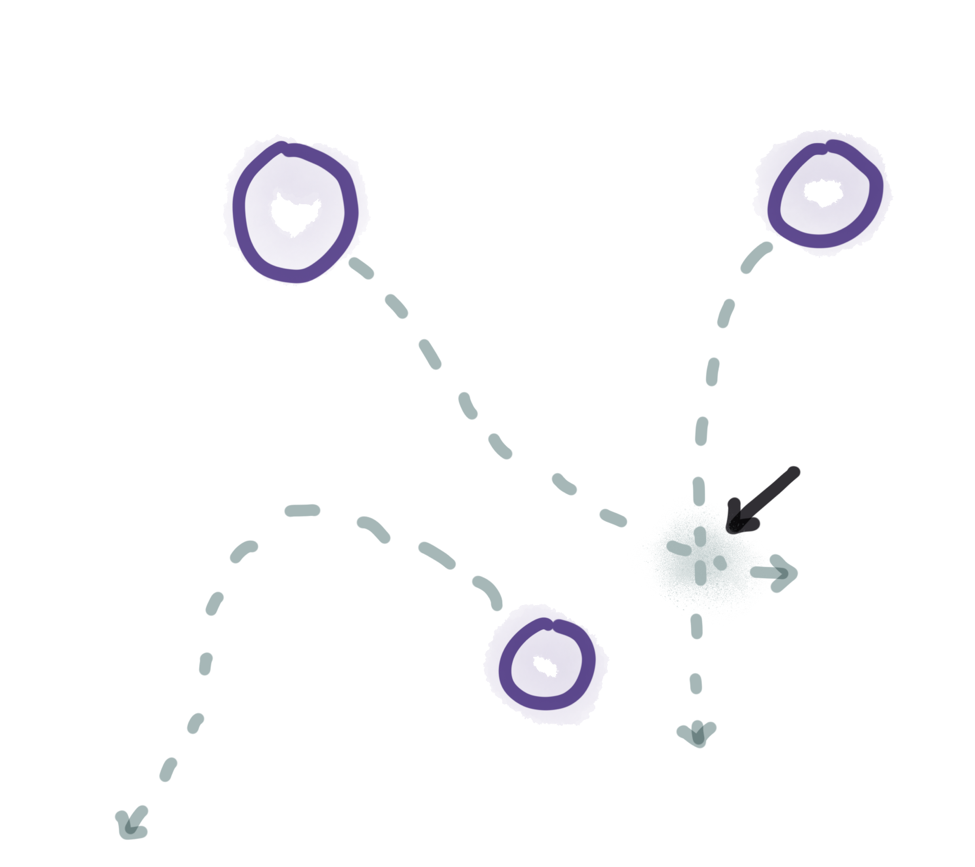## The travelling salesman problem

Because quantum algorithms generally involve some process in which all possible solutions are tried out and the correct one is then selected by amplitude amplification, quantum computers seem like they should be excellent at solving optimisation problems. To date there is no universal quantum optimisation algorithm, but there are attractive quantum solutions for some subclasses of optimisation problems. One of the most famous optimisation problems is known as the travelling salesman problem. It describes the challenge of finding the shortest route for a salesman who must travel between a number of locations. As the number of locations increases, so does the difficulty of finding an optimum route. The travelling salesman problem is NP-hard and scales exponentially with the number of locations. Precise solutions to the salesman problem can take many years (or even millions of years) to compute, even for tens of cities.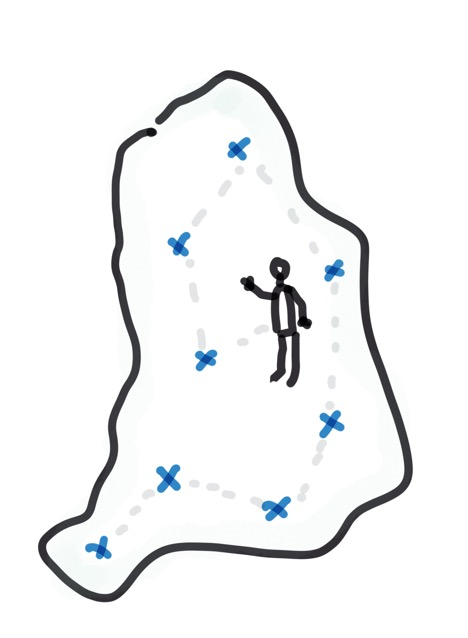The Travelling Salesman problem is an area of continuing research. Until recently, the best known quantum algorithm was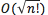(a quadratic speedup), while the best known classical algorithm was O(2n). In March 2017, an quantum algorithm was published which gave a near-quadratic speedup for many cases, and it's possible further improvements may still be discovered.

## Machine Learning

With the fields of both quantum computing and machine learning heating up in the past few years, it’s natural to ask if they can be combined. The answer, so far, appears to be a tentative ‘yes’. At a mathematical level, both quantum state calculations and machine learning models rely heavily on matrices. Conceptually, machine learning is about finding patterns in data, and the interfering-and-amplifying-waves model of quantum computation is well suited to finding patterns.

Quantum computers can do some kinds of matrix inversion with logarithmic resources (a matrix inversion is equivalent to solving a linear system of equations). This matrix inversion is useful for a range of machine learning problems. More generically, Grover’s algorithm can be used to search for the ‘right’ answer (that is, one which satisfies a given function). Other machine learning problems, such as clustering, have uniquely quantum algorithms.

Quantum computers have been theoretically proven to be better at solving what’s known as a ‘black box problem’ (discovering an unknown, random, string of bits by probing it), and researchers have demonstrated an implementation of the black box for 5 bits. As well as being able to (in principle, with a big enough computer) discover sequences so long that a classical computer would run out of time before it worked them out, quantum computers are more tolerant to noise in the readings.

### No Universal Quantum Speedup

All of these algorithms are targeted at specific classes of problems. Some, such as search, or the traveling salesman problem, have many potential applications, but the algorithms themselves are very specific. There isn’t yet a known ‘universal quantum speedup’. Put another way, in terms of the complexity classes, it’s known that BQP overlaps with NP, but it’s not yet known how much overlap there is. It seems likely that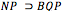, but this has not yet been proven. Peter Shor has pointed out that for a quantum algorithm to give an interesting speedup, the computational paths to the right answer need to interfere constructively, and the paths to the wrong answer need to cancel each other out. There is not yet a generalisable making-wrong-paths-cancel-each-other-out process. Given how hard the problem is, there probably never will be.

The lack of a general quantum algorithm shouldn’t detract from how interesting the known algorithms are, though, or how broadly applicable they are. The next article in this series will discuss what some of these problem domains are, and have a look into what the future might bring for quantum computation.

This is part two of a series on quantum computing. Part One, which provides an introduction to the topic and focuses on quantum computation, can be found at “Cats, Qubits, and Teleportation: The Spooky World of Quantum Computation (Part One)”. Part three, which focuses on quantum applications can be found at “Cats, Qubits, and Teleportation: The Spooky World of Quantum Computation Applications (Part 3)Holly Cummins is a full-stack developer, and cloud computing technical lead. She is also a frequent speaker, Java Champion, and author of Enterprise OSGi in Action. Holly holds a DPhil (PhD) in Quantum Computation from the University of Oxford.

Style

## Hello stranger!

You need to Register an InfoQ account or or login to post comments. But there's so much more behind being registered.

Get the most out of the InfoQ experience.

Allowed html: a,b,br,blockquote,i,li,pre,u,ul,p

• ##### Thanks.

by Johann Trixir /

• ##### Thanks.

Your message is awaiting moderation. Thank you for participating in the discussion.

Very interesting. Thank you for posting :)

Allowed html: a,b,br,blockquote,i,li,pre,u,ul,p

Allowed html: a,b,br,blockquote,i,li,pre,u,ul,p

Is your profile up-to-date? Please take a moment to review and update.

Note: If updating/changing your email, a validation request will be sent

Company name:
Company role:
Company size:
Country/Zone:
State/Province/Region:
You will be sent an email to validate the new email address. This pop-up will close itself in a few moments.